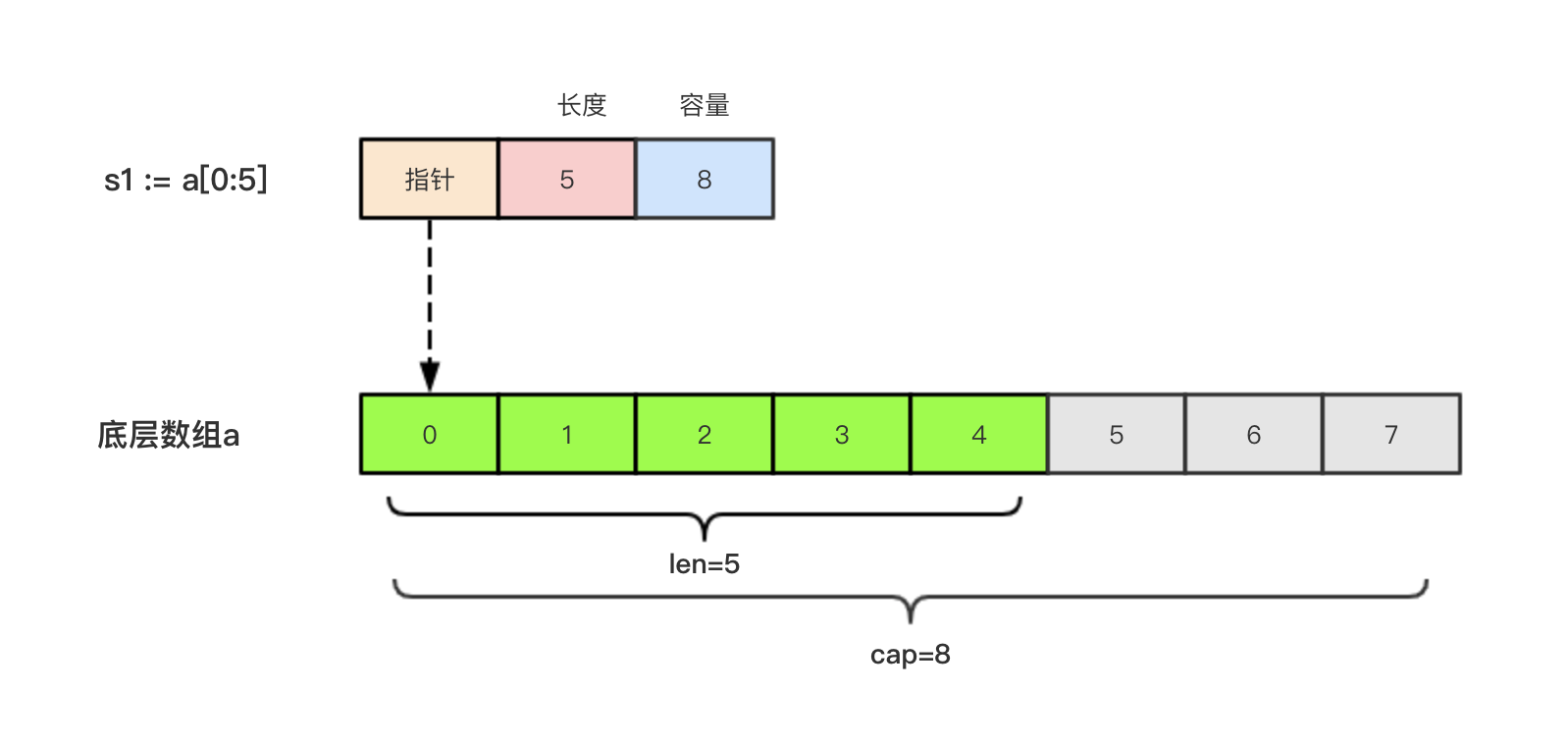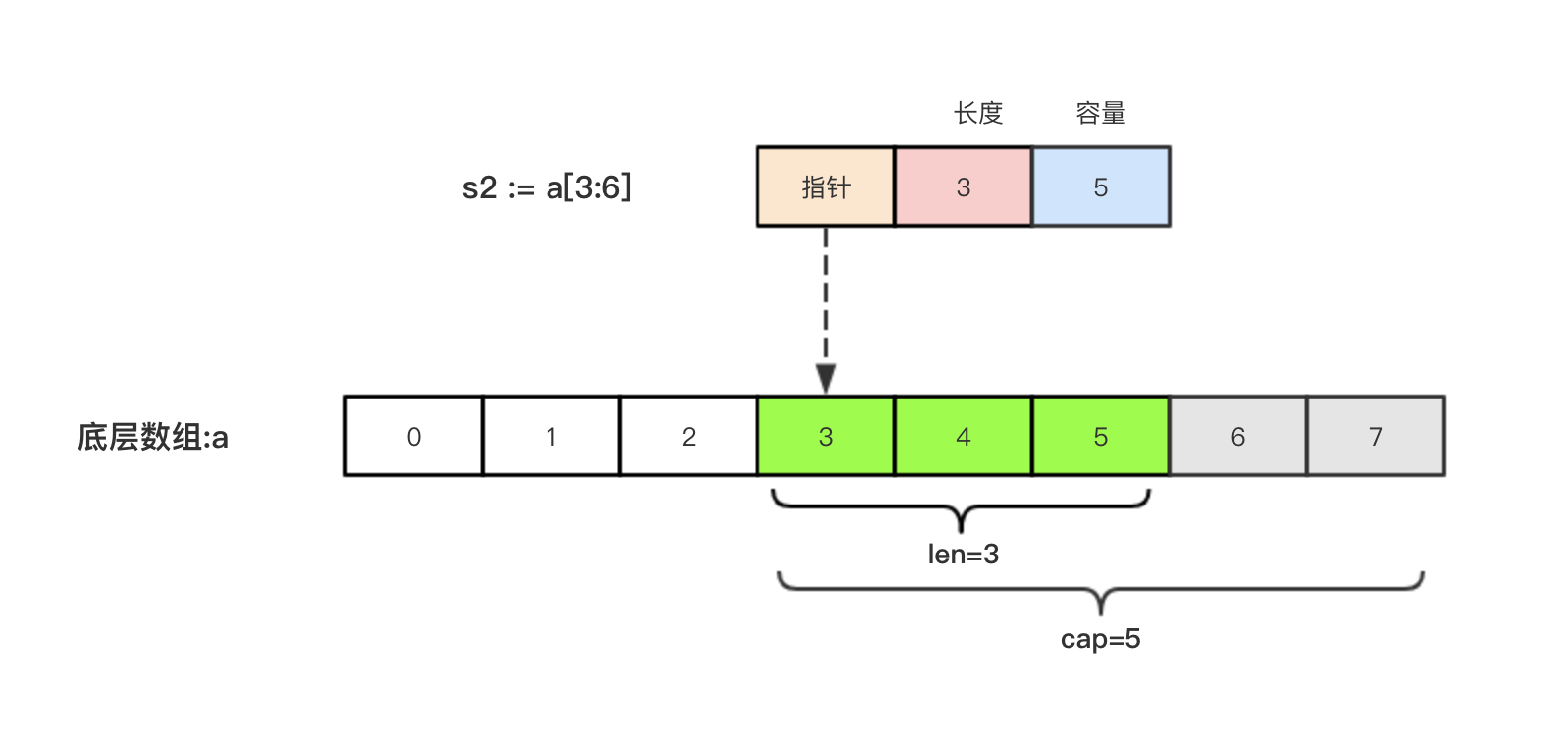## 引子

func arraySum(x int) int{
sum := 0
for _, v := range x{
sum = sum + v
}
return sum
}

a := int{1, 2, 3}

## 切片

### 切片的定义

var name []T

• name:表示变量名
• T:表示切片中的元素类型

func main() {
// 声明切片类型
var a []string              //声明一个字符串切片
var b = []int{}             //声明一个整型切片并初始化
var c = []bool{false, true} //声明一个布尔切片并初始化
var d = []bool{false, true} //声明一个布尔切片并初始化
fmt.Println(a)              //[]
fmt.Println(b)              //[]
fmt.Println(c)              //[false true]
fmt.Println(a == nil)       //true
fmt.Println(b == nil)       //false
fmt.Println(c == nil)       //false
// fmt.Println(c == d)   //切片是引用类型，不支持直接比较，只能和nil比较
}

#### 简单切片表达式

func main() {
a := int{1, 2, 3, 4, 5}
s := a[1:3]  // s := a[low:high]
fmt.Printf("s:%v len(s):%v cap(s):%v\n", s, len(s), cap(s))
}

s:[2 3] len(s):2 cap(s):4

a[2:]  // 等同于 a[2:len(a)]
a[:3]  // 等同于 a[0:3]
a[:]   // 等同于 a[0:len(a)]

func main() {
a := int{1, 2, 3, 4, 5}
s := a[1:3]  // s := a[low:high]
fmt.Printf("s:%v len(s):%v cap(s):%v\n", s, len(s), cap(s))
s2 := s[3:4]  // 索引的上限是cap(s)而不是len(s)
fmt.Printf("s2:%v len(s2):%v cap(s2):%v\n", s2, len(s2), cap(s2))
}

s:[2 3] len(s):2 cap(s):4
s2: len(s2):1 cap(s2):1

#### 完整切片表达式

a[low : high : max]

func main() {
a := int{1, 2, 3, 4, 5}
t := a[1:3:5]
fmt.Printf("t:%v len(t):%v cap(t):%v\n", t, len(t), cap(t))
}

t:[2 3] len(t):2 cap(t):4

#### 使用make()函数构造切片

make([]T, size, cap)

• T:切片的元素类型
• size:切片中元素的数量
• cap:切片的容量

func main() {
a := make([]int, 2, 10)
fmt.Println(a)      //[0 0]
fmt.Println(len(a)) //2
fmt.Println(cap(a)) //10
}

#### 切片的本质### 切片不能直接比较

var s1 []int         //len(s1)=0;cap(s1)=0;s1==nil
s2 := []int{}        //len(s2)=0;cap(s2)=0;s2!=nil
s3 := make([]int, 0) //len(s3)=0;cap(s3)=0;s3!=nil

### 切片的赋值拷贝

func main() {
s1 := make([]int, 3) //[0 0 0]
s2 := s1             //将s1直接赋值给s2，s1和s2共用一个底层数组
s2 = 100
fmt.Println(s1) //[100 0 0]
fmt.Println(s2) //[100 0 0]
}

### 切片遍历

func main() {
s := []int{1, 3, 5}

for i := 0; i < len(s); i++ {
fmt.Println(i, s[i])
}

for index, value := range s {
fmt.Println(index, value)
}
}

### append()方法为切片添加元素

Go语言的内建函数append()可以为切片动态添加元素。 可以一次添加一个元素，可以添加多个元素，也可以添加另一个切片中的元素（后面加…）。

func main(){
var s []int
s = append(s, 1)        // 
s = append(s, 2, 3, 4)  // [1 2 3 4]
s2 := []int{5, 6, 7}
s = append(s, s2...)    // [1 2 3 4 5 6 7]
}

var s []int
s = append(s, 1, 2, 3)

s := []int{}  // 没有必要初始化
s = append(s, 1, 2, 3)

var s = make([]int)  // 没有必要初始化
s = append(s, 1, 2, 3)

func main() {
//append()添加元素和切片扩容
var numSlice []int
for i := 0; i < 10; i++ {
numSlice = append(numSlice, i)
fmt.Printf("%v  len:%d  cap:%d  ptr:%p\n", numSlice, len(numSlice), cap(numSlice), numSlice)
}
}

  len:1  cap:1  ptr:0xc0000a8000
[0 1]  len:2  cap:2  ptr:0xc0000a8040
[0 1 2]  len:3  cap:4  ptr:0xc0000b2020
[0 1 2 3]  len:4  cap:4  ptr:0xc0000b2020
[0 1 2 3 4]  len:5  cap:8  ptr:0xc0000b6000
[0 1 2 3 4 5]  len:6  cap:8  ptr:0xc0000b6000
[0 1 2 3 4 5 6]  len:7  cap:8  ptr:0xc0000b6000
[0 1 2 3 4 5 6 7]  len:8  cap:8  ptr:0xc0000b6000
[0 1 2 3 4 5 6 7 8]  len:9  cap:16  ptr:0xc0000b8000
[0 1 2 3 4 5 6 7 8 9]  len:10  cap:16  ptr:0xc0000b8000

1. append()函数将元素追加到切片的最后并返回该切片。
2. 切片numSlice的容量按照1，2，4，8，16这样的规则自动进行扩容，每次扩容后都是扩容前的2倍。

append()函数还支持一次性追加多个元素。 例如：

var citySlice []string
// 追加一个元素
citySlice = append(citySlice, "北京")
// 追加多个元素
citySlice = append(citySlice, "上海", "广州", "深圳")
// 追加切片
a := []string{"成都", "重庆"}
citySlice = append(citySlice, a...)
fmt.Println(citySlice) //[北京 上海 广州 深圳 成都 重庆]

### 切片的扩容策略

newcap := old.cap
doublecap := newcap + newcap
if cap > doublecap {
newcap = cap
} else {
if old.len < 1024 {
newcap = doublecap
} else {
// Check 0 < newcap to detect overflow
// and prevent an infinite loop.
for 0 < newcap && newcap < cap {
newcap += newcap / 4
}
// Set newcap to the requested cap when
// the newcap calculation overflowed.
if newcap <= 0 {
newcap = cap
}
}
}

• 首先判断，如果新申请容量（cap）大于2倍的旧容量（old.cap），最终容量（newcap）就是新申请的容量（cap）。
• 否则判断，如果旧切片的长度小于1024，则最终容量(newcap)就是旧容量(old.cap)的两倍，即（newcap=doublecap），
• 否则判断，如果旧切片长度大于等于1024，则最终容量（newcap）从旧容量（old.cap）开始循环增加原来的1/4，即（newcap=old.cap,for {newcap += newcap/4}）直到最终容量（newcap）大于等于新申请的容量(cap)，即（newcap >= cap）
• 如果最终容量（cap）计算值溢出，则最终容量（cap）就是新申请容量（cap）。

### 使用copy()函数复制切片

func main() {
a := []int{1, 2, 3, 4, 5}
b := a
fmt.Println(a) //[1 2 3 4 5]
fmt.Println(b) //[1 2 3 4 5]
b = 1000
fmt.Println(a) //[1000 2 3 4 5]
fmt.Println(b) //[1000 2 3 4 5]
}

Go语言内建的copy()函数可以迅速地将一个切片的数据复制到另外一个切片空间中，copy()函数的使用格式如下：

copy(destSlice, srcSlice []T)

• srcSlice: 数据来源切片
• destSlice: 目标切片

func main() {
// copy()复制切片
a := []int{1, 2, 3, 4, 5}
c := make([]int, 5, 5)
copy(c, a)     //使用copy()函数将切片a中的元素复制到切片c
fmt.Println(a) //[1 2 3 4 5]
fmt.Println(c) //[1 2 3 4 5]
c = 1000
fmt.Println(a) //[1 2 3 4 5]
fmt.Println(c) //[1000 2 3 4 5]
}

### 从切片中删除元素

Go语言中并没有删除切片元素的专用方法，我们可以使用切片本身的特性来删除元素。 代码如下：

func main() {
// 从切片中删除元素
a := []int{30, 31, 32, 33, 34, 35, 36, 37}
// 要删除索引为2的元素
a = append(a[:2], a[3:]...)
fmt.Println(a) //[30 31 33 34 35 36 37]
}

Last modification：May 13th, 2020 at 03:44 pm